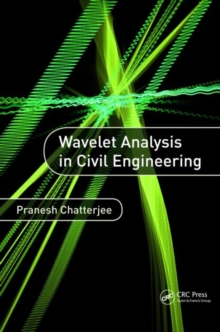Supporting your high street Find out how »
• My Account# Wavelet Analysis in Civil Engineering Hardback

#### Description

Wavelets as a Powerful Signal Processing ToolThe principles of wavelets can be applied to a range of problems in civil engineering structures, such as earthquake-induced vibration analysis, bridge vibrations, and damage identification.

This book is particularly useful for graduate students and researchers in vibration analysis, especially those dealing with random vibrations. Wavelet Analysis in Civil Engineering explains the importance of wavelets in analyzing nonstationarities in ground motions.

The example of a tank is considered to develop the problem and the model (based on linear assumptions) and several case studies are explored-fixed base, flexible base, lateral and rocking motions of foundations, with and without fluid-to explain how to account for ground motion nonstationarities.

Bridge vibrations caused by vehicle passage are explored, as is structural damage identification.

Wavelet analytic techniques starting from single degree of freedom systems to multiple degree of freedom systems are set out and detailed solutions of more complicated problems involving soil and fluid interactions are presented.

Separate chapters have been devoted to explaining the basic principles of the wavelet-based random nonstationary vibration analysis of nonlinear systems, including probabilistic analysis. Comprised of seven chapters, this text:Introduces the concept and utility of wavelet transformDescribes the discretization of ground motions using wavelet coefficientsExplains how to characterize nonstationary ground motions using statistical functionals of wavelet coefficients of seismic accelerationsDevelops the formulation of a linear single-degree-of-freedom systemShows stepwise development of the formulation of a structure idealized as a linear multi-degree-of-freedom system in terms of wavelet coefficientsDefines wavelet domain formulation of a nonlinear single-degree-of-freedom systemIntroduces the concept of probability in wavelet-based theoretical formulation of a nonlinear two-degree-of-freedom systemCovers a variety of case studies highlighting diverse applicationsWavelet Analysis in Civil Engineering explains the importance of wavelets in terms of non-stationarities of ground motions, explores the application of wavelet analytic techniques, and is an excellent resource for users addressing wavelets for the first time.

#### Information

• Format: Hardback
• Pages: 224 pages, Approx. 188 equations; 5 Tables, black and white; 90 Illustrations, black and white
• Publisher: Apple Academic Press Inc.
• Publication Date:
• Category: Calculus & mathematical analysis
• ISBN: 9781482210552

#### Other Formats

£105.00

£96.35

on all orders

###### Pick up orders

from local bookshops

£51.99

£45.59